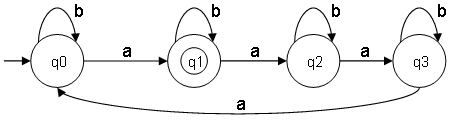# Theory of computation

The theory of computation is mathematically model a machine (for example a computer) and study the theory about it which means what are the problems which would be solved by this machine, what are the limitations of the machine etc.

## Construction of minimal DFA

Information on this page based on: YouTube: Theory Of Computation 2, Construction of minimal DFA and problems

• Construct a minimal DFA that accepts set of all strings over {a,b} of length 2
ω ∊ {a,b} and |ω| = 2

Σ = {a,b}
L = {aa,ab,ba,bb}

This DFA is NOT corect. It does accept set of all strings over {a,b} of length 2, but it should also reject strings of other lengths for example: {a,a,a}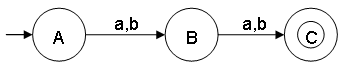This DFA is corect. It does accept set of all strings over {a,b} of length 2 and rejects strings of other lengths for example: {a,a,a}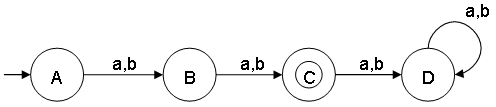When is the string accepted:
The string is accepted by the FA if we are able to reach a final state starting from the initial state upon scanning the entire string.

When is the language accepted:
The language is accepted by the FA if all strings in the language are accepted.
All strings not in the language are rejected.

Notes:
• A FA can have more than 1 final state.
• State D is also called the dead or trap state.
• This minimal DFA has 4 states and state C (also referred as q2) is the final state.
• The language L is finite which means the FA is finite (= finite number of states).

• Construct a minimal DFA that accepts set of all strings over {a,b} of length 2 or more
ω ∊ {a,b} and |ω| >= 2

L = {aa,ab,ba.bb,aaa, ..., bbb, ...}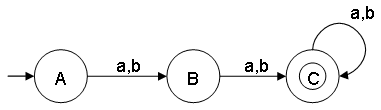Notes:
• For a language L you can draw many DFA diagrams, but there is only 1 minimal DFA.
The word "minimal" refers to the minimal number of states used.
• The language L is infinite, we do not know if the FA is finite or infinite. By drawing the FA diagram there are a finite number of states which means this minimal DFA is finite.
• State A represents set of all strings of length 0.
• State B represents set of all strings of length 1.
• State C represents set of all strings of length 2.
• This minimal DFA has 3 states and state C (also referred as q2) is the final state.

• Construct a minimal DFA that accepts set of all strings over {a,b} of length 2 or less
ω ∊ {a,b} and |ω| <= 2

L = {ε,a,b,aa,ab,ba,bb}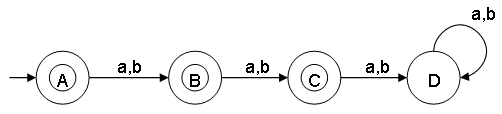Notes:
• For a language L you can draw many DFA diagrams, but there is only 1 minimal DFA.
The word "minimal" refers to the minimal number of states used.
• The language L is finite which means the FA is finite (= finite number of states).
• State A represents set of all strings of length 0.
• State B represents set of all strings of length 1.
• State C represents set of all strings of length 2.
• State D represents set of all strings of length 3 or more which will be rejected.
• If you have ε, always make the inital state a final state.
• This minimal DFA has 4 states and states A, B and C (respectively referred as q0, q1, q2) are the final states.

• Number of states required in a minimal DFA that accepts set of all strings with a certain length
In general a minimal DFA accept strings of length n requiring the following the number of states:

ω ∊ {a,b}
|ω| = n, number of states required: (n+2)
|ω| >= n, number of states required: (n+1)
|ω| <= n, number of states required: (n+2)

• Construct a minimal DFA that accepts set of all strings over {a,b}, where the length modulo 2 = 0
ω ∊ {a,b} and |ω| mod 2 = 0

L = {ε,aa,ab,ba,bb,aaaa,bbbb,...}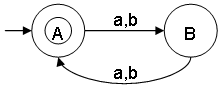Notes:
• Even length strings satisfies the condition: |ω| mod 2 = 0
• ε is a string of length 0 and |ε| mod 2 = 0
• The language L is infinite, we do not know if the FA is finite or infinite. By drawing the FA diagram there are a finite number of states which means this minimal DFA is finite.
• |ω| mod 2 = 0 can also be written as |ω| ≅ 0 (mod 2). The symbol ≅ means congruent.
• State A represents set of all strings of even lengths.
• State B represents set of all strings of odd lengths.
• This minimal DFA has 2 states and state A (also referred as q0) is the final state.

• Construct a minimal DFA that accepts set of all strings over {a,b}, where the length modulo 2 = 1
ω ∊ {a,b} and |ω| mod 2 = 1

L = {a,b,aaa,aab,...}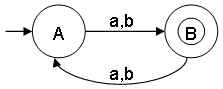Notes:
• Odd length strings satisfies the condition: |ω| mod 2 = 1
• ε is a string of length 0 and does not satisfy condition |ε| mod 2 = 1
• The language L is infinite, we do not know if the FA is finite or infinite. By drawing the FA diagram there are a finite number of states which means this minimal DFA is finite.
• |ω| mod 2 = 1 can also be written as |ω| ≅ 1 (mod 2). The symbol ≅ means congruent.
• State A represents set of all strings of even lengths.
• State B represents set of all strings of odd lengths.
• This minimal DFA has 2 states and state B (also referred as q1) is the final state.

• Construct a minimal DFA that accepts set of all strings over {a,b}, where the length modulo 3 = 0
ω ∊ {a,b} and |ω| mod 3 = 0

L = {ε,aaa,aab,aaaaaa,...}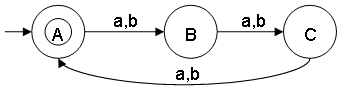Notes:
• Strings with lengths 0, 3, 6, 9, ... satisfies the condition: |ω| mod 3 = 0
• ε is a string of length 0 and does satisfy condition |ε| mod 3 = 0
• The language L is infinite, we do not know if the FA is finite or infinite. By drawing the FA diagram there are a finite number of states which means this minimal DFA is finite.
• |ω| mod 3 = 0 can also be written as |ω| ≅ 0 (mod 3). The symbol ≅ means congruent.
• State A represents set of all strings of length 0, 3, 6, 9, ....
• This minimal DFA has 3 states and state A (also referred as q0) is the final state.

• Construct a minimal DFA that accepts set of all strings over {a,b}, where the length modulo 3 = 1
ω ∊ {a,b} and |ω| mod 3 = 1

L = {a,b,aaaa,aaab,...}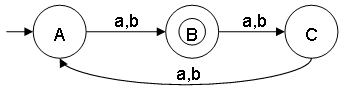Notes:
• Strings with lengths 1, 4, 7, 10, ... satisfies the condition: |ω| mod 3 = 1
• ε is a string of length 0 and does not satisfy condition |ε| mod 3 = 1
• The language L is infinite, we do not know if the FA is finite or infinite. By drawing the FA diagram there are a finite number of states which means this minimal DFA is finite.
• |ω| mod 3 = 1 can also be written as |ω| ≅ 1 (mod 3). The symbol ≅ means congruent.
• State B represents set of all strings of length 1, 4, 7, 10, ....
• This minimal DFA has 3 states and state B (also referred as q1) is the final state.

• How many states are in a minimal DFA that accepts set of all strings over {a,b}, where the length modulo n = 0
ω ∊ {a,b} and |ω| mod n = 0

Answer: The number of states is n

For example:
ω ∊ {a,b} and |ω| mod 2 = 0 -> number of states = 2
ω ∊ {a,b} and |ω| mod 3 = 0 -> number of states = 3
ω ∊ {a,b} and |ω| mod 4 = 0 -> number of states = 4
ω ∊ {a,b} and |ω| mod 5 = 0 -> number of states = 5
ω ∊ {a,b} and |ω| mod 6 = 0 -> number of states = 6
:

• Construct a minimal DFA that accepts set of all strings over {a,b}, where the number of "a" are 2
ω ∊ {a,b} and na(ω) = 2

L = {aa,baa,aab,aba,aabb,bbaa,bbbaa,...}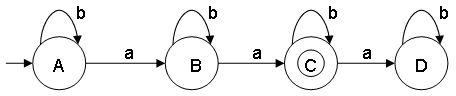Notes:
• The language L is infinite, we do not know if the FA is finite or infinite. By drawing the FA diagram there are a finite number of states which means this minimal DFA is finite.
• State A represents set of all strings of length 0
• State B represents set of all strings of length 1
• State C represents set of all strings of length 2
• State D (dead state) represents set of all strings of length 3
• This minimal DFA has 4 states and state C (also referred as q2) is the final state.

• Construct a minimal DFA that accepts set of all strings over {a,b}, where the number of "a" are at least 2
ω ∊ {a,b} and na(ω) >= 2

L = {aa,aaa,baa,aab,aba,aaab,aabb,bbaa,bbbaa,aaabb,...}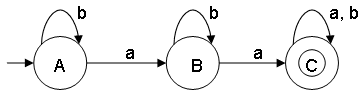Notes:
• The language L is infinite, we do not know if the FA is finite or infinite. By drawing the FA diagram there are a finite number of states which means this minimal DFA is finite.
• State A represents set of all strings of length 0
• State B represents set of all strings of length 1
• State C represents set of all strings of length 2
• This minimal DFA has 3 states and state C (also referred as q2) is the final state.

• Construct a minimal DFA that accepts set of all strings over {a,b}, where the number of "a" is 2 or less
ω ∊ {a,b} and na(ω) <= 2

L = {ε,a,b,aa,ab,ba,bb,aab,aba,baa,aabb,aabbb,...}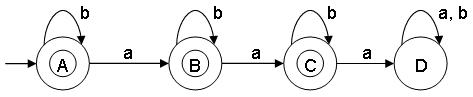Notes:
• The language L is infinite, we do not know if the FA is finite or infinite. By drawing the FA diagram there are a finite number of states which means this minimal DFA is finite.
• State A represents set of all strings of length 0
• State B represents set of all strings of length 1
• State C represents set of all strings of length 2
• State D (dead state) represents set of all strings of length 3
• This minimal DFA has 4 states and state A, B and C (respectively referred as q0, q1 and q2) are the final states.

• Construct a minimal DFA that accepts set of all strings over {a,b}, where the number of "a" is even
ω ∊ {a,b} and na(ω) mod 2 = 0

L = {ε,b,aa,aab,aba,baa,aaaab,aababab,...}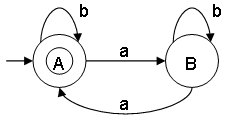Notes:
• The language L is infinite, we do not know if the FA is finite or infinite. By drawing the FA diagram there are a finite number of states which means this minimal DFA is finite.
• na(ω) mod 2 = 0 can also be written as na(ω) ≅ 0 (mod 2). The symbol ≅ means congruent.
• State A represents set of all strings of length 0, 2, 4, 6, 8,... (even lengths)
• State B represents set of all strings of length 1, 3, 5, 7, 9,... (odd lengths)
• This minimal DFA has 2 states and state A (also referred as q0) is the final state.

• Construct a minimal DFA that accepts set of all strings over {a,b}, where the number of "a" is odd
ω ∊ {a,b} and na(ω) mod 2 = 1

L = {a,ab,abb,abaa,aaab,aabaaa,ababab,...}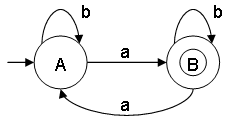Notes:
• The language L is infinite, we do not know if the FA is finite or infinite. By drawing the FA diagram there are a finite number of states which means this minimal DFA is finite.
• na(ω) mod 2 = 1 can also be written as na(ω) ≅ 1 (mod 2). The symbol ≅ means congruent.
• State A represents set of all strings of length 0, 2, 4, 6, 8,... (even lengths)
• State B represents set of all strings of length 1, 3, 5, 7, 9,... (odd lengths)
• This minimal DFA has 2 states and state B (also referred as q1) is the final state.

• Construct a minimal DFA that accepts set of all strings over {a,b}, where the number of "a" modulo 3 = 0
ω ∊ {a,b} and na(ω) mod 3 = 0

L = {ε,aaa,aaba,aaab,aaabb,aaaaaa,...}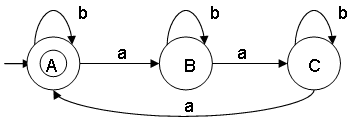Notes:
• Strings with lengths 0, 3, 6, 9, ... satisfies the condition: na(ω) mod 3 = 0
• ε is a string of length 0 and does satisfy condition na(ω) mod 3 = 0
• The language L is infinite, we do not know if the FA is finite or infinite. By drawing the FA diagram there are a finite number of states which means this minimal DFA is finite.
• na(ω) mod 3 = 0 can also be written as na(ω) 0 (mod 3). The symbol ≅ means congruent.
• State A represents set of all strings where the length of "a" are 0, 3, 6, 9, ....
• This minimal DFA has 3 states and state A (also referred as q0) is the final state.

• Which state is the final state in a minimal DFA with N states that accepts set of all strings over {a,b}, where the number of "a" modulo N = k
ω ∊ {a,b} and na(ω) mod N = k and N = number of states

Number of states = N and ω ∊ {a,b} and na(ω) mod N = k -> final state = qk

Number of states = N and ω ∊ {a,b} and na(ω) mod N = 0 -> final state = q0
Number of states = N and ω ∊ {a,b} and na(ω) mod N = 1 -> final state = q1
Number of states = N and ω ∊ {a,b} and na(ω) mod N = 2 -> final state = q2
Number of states = N and ω ∊ {a,b} and na(ω) mod N = 3 -> final state = q3
:

For example:
Number of states = 3 and k = 2
ω ∊ {a,b} and na(ω) mod 3 = 2

L = {aa,aaaaa,aaaaaaaa,aabb,baaaaa,...}
Final state = q2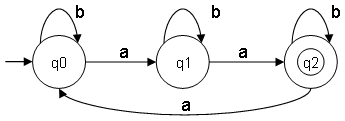For example:
Number of states = 4 and k = 1
ω ∊ {a,b} and na(ω) mod 4 = 1

L = {a,aaaaa,aaaaaaaaa,ab,aabaaa,...}
Final state = q1